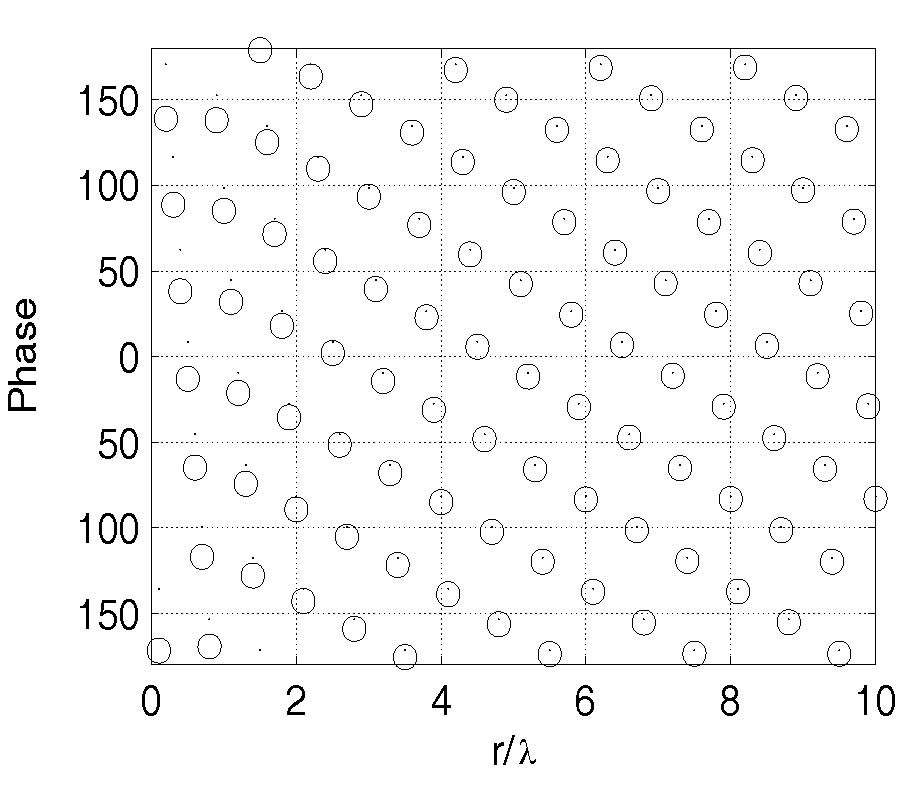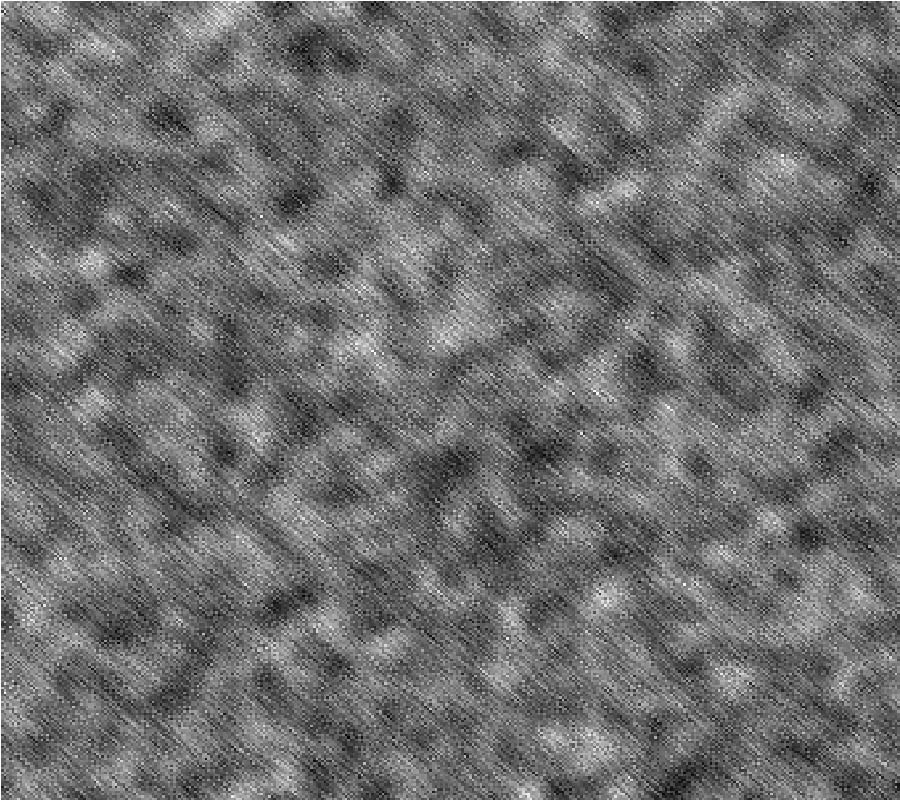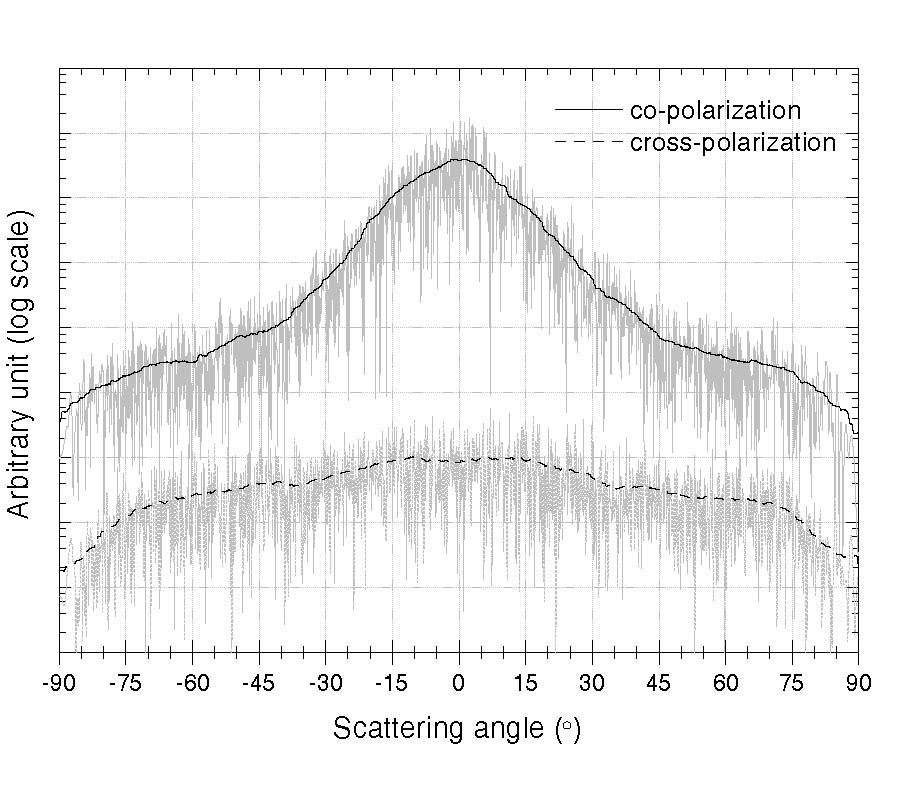ISSN: 1070-4698

# Vol. 37

Latest Volume
All Volumes
All Issues

PIER Vol. 37, 319-343, 2002. doi:10.2528/PIER02080106

###Model of Electromagnetic Wave Scattering from Sea Surface with and Without Oil Slicks

Alla I. Timchenko and Klaus Schuenemann

The problem of scattering from sea surface covered by oil films is investigated by using a composite random rough surface model. A model is developed which extends the range of validity beyond the small perturbation theory. A general expression for the scattering cross section is obtained taking into account a modulation of the rough surface by long surface waves. A numerical study for the radar scattering cross section is provided in order to investigate the influence of the different ranges of the rough surface spectrum on the backscattering depression. For the case of backscattering, the contrast of radar signals scattered from a slick-free and a slick-covered surface is evaluated. The study is also carried through for two-frequency probing. A possibility to explain the mechanism of the depression of backscattering is discussed. The results of this study demonstrate the importance of the improved model which takes into account the entire spectrum of the sea surface roughness for the description of scattering from an ocean surface with and without oil slicks.

PIER Vol. 37, 289-317, 2002. doi:10.2528/PIER02080502

###Scattering of a Plane Wave by 1-Dimensional Dielectric Rough Surfaces Study of the Field in a Nonorthogonal Coordinate System

Richard Dusséaux and C. Baudier

We present a rigorous method giving the field scattered by a dielectric plane surface with a local cylindrical perturbation illuminated by a plane wave. The theory is based on Maxwell's equations written in a nonorthogonal coordinate system. A Method of Moments (PPMoM) with Pulses for basis and weighting functions is applied for solving in the spectral domain. For several deterministic profiles, we study the influence of polarization, incidence angle and perturbation depth and show that the distance defining the far-field approximation depends on the observation angle.

PIER Vol. 37, 251-287, 2002. doi:10.2528/PIER02070603

###Small-Slope Approximation Method: a Further Study of Vector Wave Scattering from Two-Dimensional Surfaces and Comparison with Experimental Data

Gerard Berginc

This paper deals with the calculation of the scattering cross-section of polarized electromagnetic plane waves from 2-D metallic and dielectric randomly rough surfaces. The scattering crosssection of object is calculated by the Local Small Slope Approximation (SSA), the scattering cross-section is then compared with experimental data. In this paper, second order terms of the SSA method have been numerically implemented in order to obtain accurate results for a large range of slope. In this paper, we consider scattered and incident wave vectors in arbitrary directions, metallic and dielectric materials with complexp ermittivity. Surfaces are considered with Gaussian probability density functions for surface heights and Gaussian or non-Gaussian correlation functions. The coherent and incoherent components of the electromagnetic intensity for cross- and co-polarization are calculated in the bistatic case and we give several comparisons of the theory with measured data.

PIER Vol. 37, 235-250, 2002. doi:10.2528/PIER02032100

###Scattering of a Plane Wave by Rough Surfaces: a New Curvilinear Coordinate System Based Approach

Gerard Granet, K. Edee, and Didier Felbacq

We present a curvilinear coordinate based method giving the field scattered by a plane surface with a cylindrical local perturbation illuminated by a plane wave. The boundary-value problem turns on the same scalar eigen equation that is solved in the spectral domain. Numerical results are successfully compared with those obtained by other rigorous methods

PIER Vol. 37, 221-234, 2002. doi:10.2528/PIER02042601

###Study on the Two-Frequency Scattering Cross Section and Pulse Broadening of the One-Dimensional Fractal Sea Surface at Millimeter Wave Frequency

Cheol Kim

Based on the Kirchhoff approximation for the surfaces with small slopes, the pulse beam wave scattering fromthe onedimensional fractal sea surface with the actual spectrum is studied. The influence of the different fractal dimension, incident angle, and the center frequency on the distributions of the two-frequency scattering cross section is analyzed. The numerical result shows that there exists the largest coherence bandwidth for the two-frequency scattering cross section at the specular direction. The coherence bandwidth will increase with the decrease of the fractal dimension and with the increase of the incident angle and the center frequency, as well. It is also found that the scattering power takes a pulse shape, but with a pulse broadening for the incident power being δ function, this pulse broadening is inversely proportional to the coherence bandwidth.

PIER Vol. 37, 205-219, 2002. doi:10.2528/PIER02101000

###Two-Scale Polarimetric Emissivity Model: Efficiency Improvements and Comparisons with Data

David Lyzenga and John F. Vesecky

The two-scale model provides a framework for explaining the polarization and angular dependence of the microwave radiation emitted from the ocean surface. In this model the surface is viewed as a collection of randomly oriented facets. The emissivity of each facet is calculated using the small perturbation method (SPM), and that of the entire surface is obtained by integrating the local emissivity over all possible surface slopes, weighted by the probability of encountering these slopes. Since each SPM calculation involves a double integral, the model requires in principle the evaluation of a fourdimensional integral. This paper explores two methods for reducing the computational time required by the two-scale model. In one version, the azimuthal dependence of the local emissivity is represented by a truncated Fourier series and slope integral is computed numerically. In the second version the slope integral is carried out analytically, after expanding the integrand as a Taylor series in the surface slope. Hydrodynamic modulation effects are included in order to explain the upwind-downwind asymmetry of the emissivity. The calculated emissivities from the two versions of the model are compared with each other and with airborne and spaceborne measurements.

PIER Vol. 37, 191-203, 2002. doi:10.2528/PIER02031506

###A Semi-Empirical Algorithm of Water Transparency at the Green Wavelength Band of Optical Remote Sensing

Jouni Pulliainen and Martti Hallikainen

This study employed water transparent characteristics from the Gulf and archipelago of Finland and the corresponding data sets of optical sensors at the green wavelength band to estimate Secchi disk depth (SDD). The SDD is one major optical measurement of water transparency in the study area,where the coastal waters are dominated by absorption from both dissolved and particulate organic matter since the Gulf is optically dominated by scattering from suspended sediments. Concurrent in situ measurements,Landsat TM and simulated SeaWiFS data were obtained in August 1997. The results show that the SDD from the narrow green bandwidth (20 nm) data of simulated SeaWiFS is slightly better than the SDD from the broad green bandwidth (80 nm) data of TM using the semi-empirical algorithm developed in the study. The study of water transparent characteristics in the area still needs to be further investigated using SeaWiFS,MODIS and MERIS in the future.

PIER Vol. 37, 143-190, 2002. doi:10.2528/PIER02040800

###A Composite Model for Estimation of Polarimetric Thermal Emission from Foam-Covered Wind-Driven Ocean Surface

Jin Au Kong

This paper presents theoretical studies of polarimetric thermal emission from foam-covered ocean surface based on a composite volume and rough surface scattering model using the radiative transfer theory. The sea foam is modeled as a layer containing randomly distributed thin-film water bubbles. The small perturbation method (SPM) is used for random rough ocean surface, where the bistatic scattering is calculated up to the second order. The radiative transfer equations with a rough interface are solved using an iterative technique. Model predictions are compared with empirical expressions for foam emissivity and with the WINDRAD measurement data.

PIER Vol. 37, 129-141, 2002. doi:10.2528/PIER02010100

###Mathematical Modeling of Electromagnetic Wave Scattering by Wavy Periodic Boundary Between Two Media

Jean Chandezon, Anatoly Poyedinchuk, Yury A. Tuchkin, and Nataliya Yashina

The extension of C method, combined with idea of Tikhonov's regularization is proposed. The regularizing algorithm for numerical solution of electromagnetic wave diffraction by the boundary of dielectric media is developed. This algorithm is based on the solution of the system linear algebraic equations of C method as subject of regularizing method of A. N. Tikhonov. The numerical calculations of scattered field in the case of E-polarization are presented. The efficiency and reliability of the method for the solution of the problems of boundary shape reconstruction have been proved and demonstrated numerically for several situations.

PIER Vol. 37, 101-128, 2002. doi:10.2528/PIER01111800

###Modelization of the Scattering of Electromagnetic Waves from the Ocean Surface

Gabriel Soriano

In this paper, two models for the solution of the electromagnetic bistatic scattering from sea surface are suggested. A rigorous formalism leading to weakly singular integral equations is presented, as well as the surface impedance approximation for low penetrable media and the beam simulation method to synthesize incident beams with arbitrary size. This rigorous integral method is used to test first order approximations, and it is shown that the Small Slope Approximation is very accurate in predicting the scattering crosssection fromthe high spatial frequencies of the sea surface. This result led us to suggest an improvement of the classical two-scale model, consisting in replacing the small perturbation theory by the small slope approximation. This change allows the cut-off spatial frequency to be shifted so that the use of geometrical Optics is restricted to the large scales.

PIER Vol. 37, 79-99, 2002. doi:10.2528/PIER02013000

###Higher Order Emission Model Study of BI-Sinusoidal Surface Brightness Temperatures

Joel Johnson

Models for microwave thermal emission from a rough surface are currently of interest due to the goal of improved sea surface wind vector retrievals from polarimetric brightness temperature measurements. Models based on either a small slope approximation or on a physical optics approach have been proposed and have shown some success in matching observations. Both of these models involve series solutions, but computation of higher order terms typically is difficult, particularly for multi-scale sea surface models. Knowledge of higher order term contributions, however, would assist in understanding the limitations of the low-order methods applied in practice. In this paper, higher order results from both the small slope and physical optics methods are studied and compared for a simple bi-sinusoidal surface model (i.e. height profile = Asin(2πx/Px) sin(2πy/Py), where Px and Py are the surface periods in the x and y directions, respectively). Results show both methods to provide good predictions for moderate slope "large scale" surfaces (i.e. periods large compared to the observing electromagnetic wavelength) when shadowing and multiple scattering effects are negligible, while only the small slope theory correctly predicts emission from "small scale" profiles. The influence of both shadowing and multiple scattering effects is examined, and the "binary" shadowing behavior used in the physical optics method is suggested as a source of larger errors observed as shadowing effects increase.

PIER Vol. 37, 31-78, 2002. doi:10.2528/PIER01100801

###Microwave Analytical Backscattering Models from Randomly Rough Anisotropic Sea Surface --- Comparison with Experimental Data in C and Ku Bands

Christophe Bourlier and Gerard Berginc

The small slope approximation (SSA) and the Kirchhoff approach (KA) are applied to the prediction of microwave sea surface backscatter for both Ku and C bands for various wind speeds and incident angles. Numerical results are obtained assuming a non-directional surface wavenumber spectrum and compared with azimuthally averaged C- and Ku-band radar backscattering data. The KA can be obtained rigorously for a perfectly-conducting surface, whereas for a dielectric surface, either the KA of order one (KA1) or the stationary phase (SP) method can be used. Numerical results are obtained assuming a non-directional surface wavenumber spectrum and compared with azimuthally C and Ku bands radar backscattering data for incidence angles of interest for remote sensing. Since the SSA and KA formulations are expressed in polar coordinates, the backscattering coefficient is expressed in terms of surface height autocorrelation and its derivatives of one- and second- orders computed from integrating the sea spectrum multiplied by Bessel functions of the first kind. This allows to have for KA and first-order SSA (SSA-1), a single numerical integration over the radial distance instead of four, when the cartesian coordinates is chosen. Moreover, the azimuthal harmonic magnitudes of the backscattering coefficient according to the wind direction can be performed separately. For an isotropic sea surface assumed to be perfectly conducting where the KA is valid, the deviation between SSA and KA models is smaller than the one computed from the SP model for HH polarization. For the VV polarization, the difference is greater, since the polarization term of SSA is given by the small perturbation method, whereas for the KA approach, it is equal to the Fresnel coefficient. For an anisotropic sea surface, the comparison of KA with SSA-1 leads to the same conclusion. The isotropic part and the second azimuthal harmonic of the backscattering coefficient are also compared with empirical backscattering models CMOD2-I3 and SASS-II valid in C and Ku bands, respectively.

PIER Vol. 37, 1-30, 2002. doi:10.2528/PIER01100800

###Polarimetric Emission Model of the Sea at Microwave Frequencies and Comparison with Measurements

Peter W. Gaiser

At wo-scale scattering model of the sea developed in terms of wind-generated stochastic processes of the surface-the elevation spectral density of the small-scale structure and the probability density of slopes of the large scale roughness-is combined with the Durden/Vesecky  wave height spectral model to analyze recent polarimetric measurements. Ad hoc parameter values are found for the wave model that allow the two-scale model to account for essentially all of the azimuthal features, amplitude and phase, appearing in all four Stokes parameters for the Jet Propulsion Laboratory (JPL) aircraft measurements at 19.35 and 37 GHz  and recent Naval Research Laboratory (NRL) aircraft measurements at 10.7 GHz. The excellent agreement provides support for the validation of the approximations of the two-scale model for the range of conditions encountered. The ad hoc parameters of the wave model are developed using the 19.35 and 37.0 GHz data and then tested with 10.7 GHz data. The twoscale model should be useful in studies dealing with simulations and retrievals of surface wind direction from satellite-based polarimetric measurements.